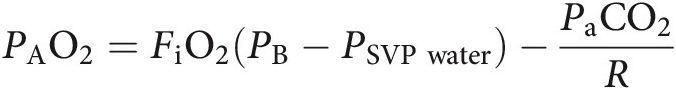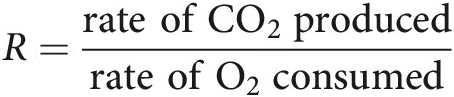# Chapter 18 – Alveolar Gas Equation

## Abstract

The partial pressure gradient of O2 between the alveolus and the pulmonary capillaries is one of the key factors that determine the rate of O2 diffusion across the alveolar–capillary barrier (see Chapter 10). Unfortunately, it is not possible to directly measure the PAO2. Instead, PAO2 can be estimated using the alveolar gas equation (AGE).

## Chapter 18 Alveolar Gas Equation

### Can you measure the partial pressure of oxygen in the alveolus?

The partial pressure gradient of O2 between the alveolus and the pulmonary capillaries is one of the key factors that determine the rate of O2 diffusion across the alveolar–capillary barrier (see Chapter 10). Unfortunately, it is not possible to directly measure the PAO2. Instead, PAO2 can be estimated using the alveolar gas equation (AGE).

### What is the AGE?

The AGE1 allows PAO2 to be estimated from variables that are easily measured.

## Key equation: simplified AGE

PAO2=FiO2PB−PSVPwater−PaCO2Rwhere FiO2 for dry air is 20.93%; PB at sea level is 101.325 kPa. Inspired air becomes fully saturated with water vapour by the time it reaches the carina. PSVP water is the saturated vapour pressure of water, which at body temperature is 6.3 kPa. PaCO2 is measured in kilopascals. R is the respiratory quotient, usually taken as 0.8.

The AGE tells us that PAO2 is essentially dependent on three variables:

• The inspired fraction of O2. According to the AGE, increasing FiO2 will result in a greater PAO2, thus increasing the pressure gradient across the alveolar–capillary barrier; the rate of O2 diffusion will increase (Fick’s law – see Chapter 10).

• Barometric pressure. PB decreases exponentially with ascent to altitude (see Chapter 87). According to the AGE, as the elevation above sea level increases, the fall in PB results in a lower PAO2 and thus a reduced rate of O2 diffusion across the alveolar–capillary barrier.

• Alveolar ventilation. As PaCO2 is inversely proportional to A (see Chapter 11):

1. An increase in A (hyperventilation) results in a decrease in PaCO2. According to the AGE, PAO2 will increase, thus increasing the rate of O2 diffusion across the alveolar–capillary barrier.

2. A decrease in A (hypoventilation) results in an increase in PaCO2. According to the AGE, PAO2 will decrease, thus reducing the rate of O2 diffusion across the alveolar–capillary barrier.

### What is the respiratory quotient? Why does it differ for different dietary substrates?

The respiratory quotient R is the ratio of CO2 production and O2 consumption:

R=rate ofCO2producedrate ofO2consumed